Скачать презентацию A Systematic Procedure for Analysis and Modification of

465599905530a81cdbf8c3a028bcac42.ppt

• Количество слайдов: 28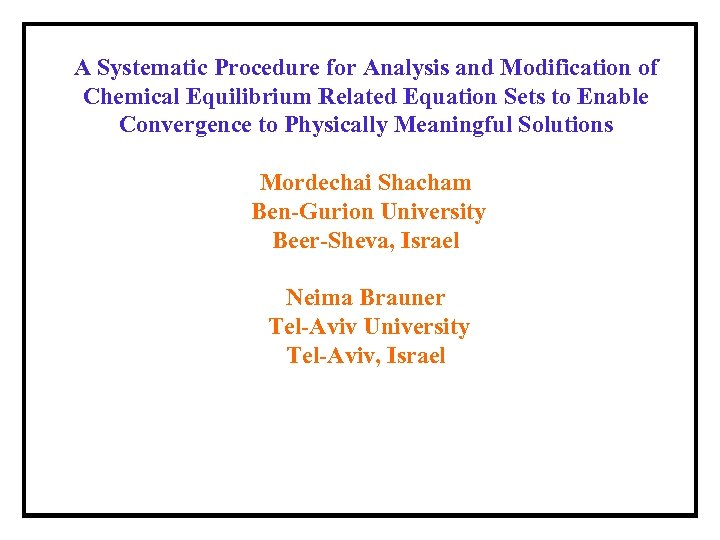A Systematic Procedure for Analysis and Modification of Chemical Equilibrium Related Equation Sets to Enable Convergence to Physically Meaningful Solutions Mordechai Shacham Ben-Gurion University Beer-Sheva, Israel Neima Brauner Tel-Aviv University Tel-Aviv, Israel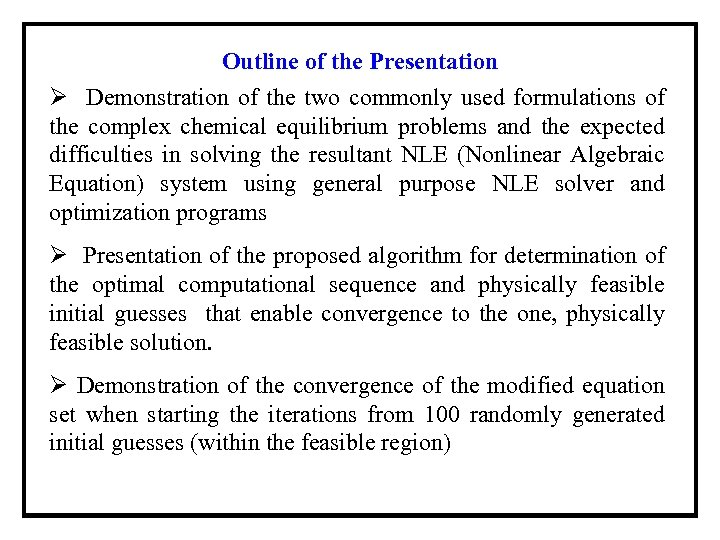Outline of the Presentation Ø Demonstration of the two commonly used formulations of the complex chemical equilibrium problems and the expected difficulties in solving the resultant NLE (Nonlinear Algebraic Equation) system using general purpose NLE solver and optimization programs Ø Presentation of the proposed algorithm for determination of the optimal computational sequence and physically feasible initial guesses that enable convergence to the one, physically feasible solution. Ø Demonstration of the convergence of the modified equation set when starting the iterations from 100 randomly generated initial guesses (within the feasible region)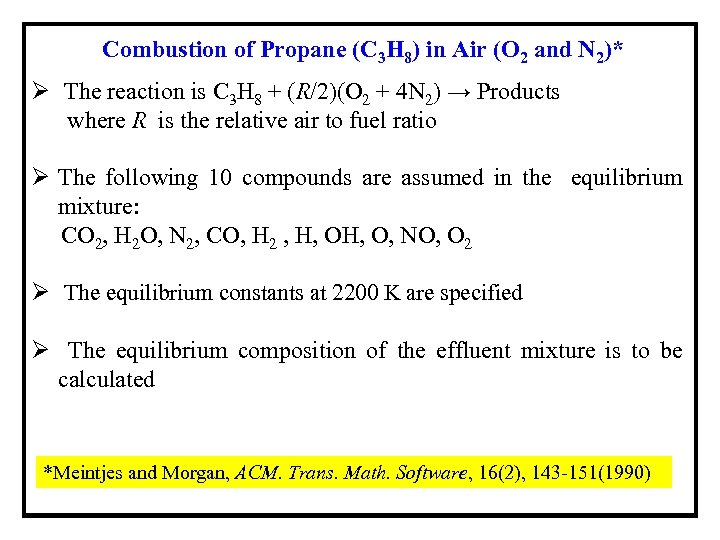Combustion of Propane (C 3 H 8) in Air (O 2 and N 2)* Ø The reaction is C 3 H 8 + (R/2)(O 2 + 4 N 2) → Products where R is the relative air to fuel ratio Ø The following 10 compounds are assumed in the equilibrium mixture: CO 2, H 2 O, N 2, CO, H 2 , H, O, NO, O 2 Ø The equilibrium constants at 2200 K are specified Ø The equilibrium composition of the effluent mixture is to be calculated *Meintjes and Morgan, ACM. Trans. Math. Software, 16(2), 143 -151(1990)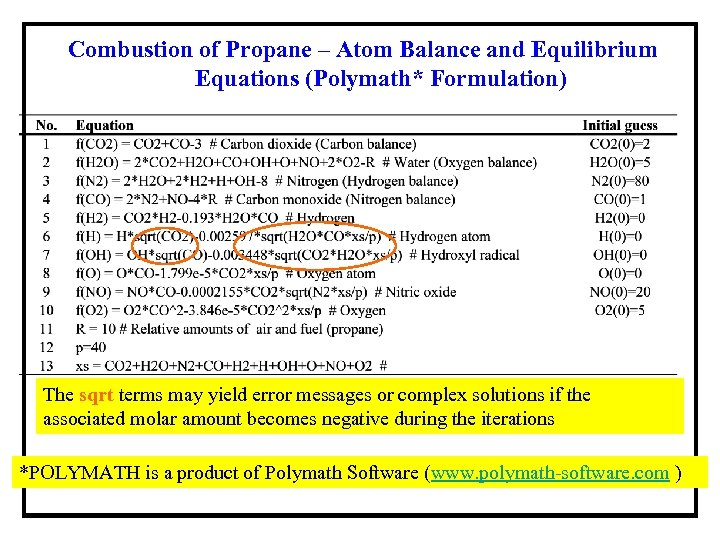Combustion of Propane – Atom Balance and Equilibrium Equations (Polymath* Formulation) The sqrt terms may yield error messages or complex solutions if the associated molar amount becomes negative during the iterations *POLYMATH is a product of Polymath Software (www. polymath-software. com )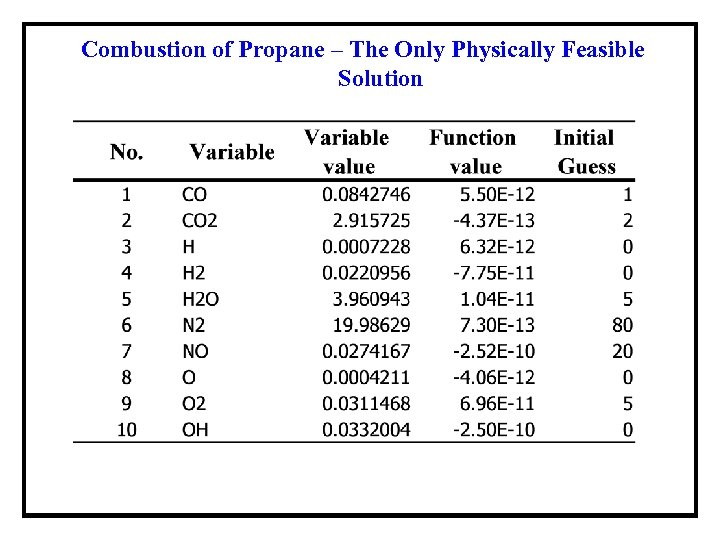Combustion of Propane – The Only Physically Feasible Solution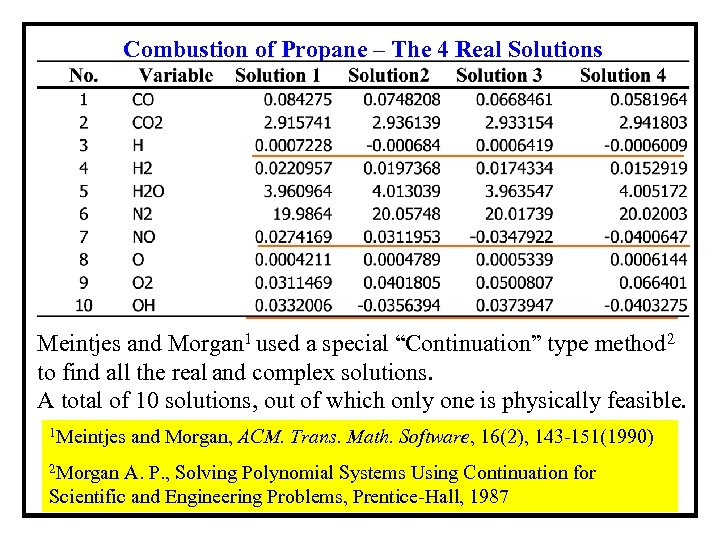Combustion of Propane – The 4 Real Solutions Meintjes and Morgan 1 used a special “Continuation” type method 2 to find all the real and complex solutions. A total of 10 solutions, out of which only one is physically feasible. 1 Meintjes 2 Morgan and Morgan, ACM. Trans. Math. Software, 16(2), 143 -151(1990) A. P. , Solving Polynomial Systems Using Continuation for Scientific and Engineering Problems, Prentice-Hall, 1987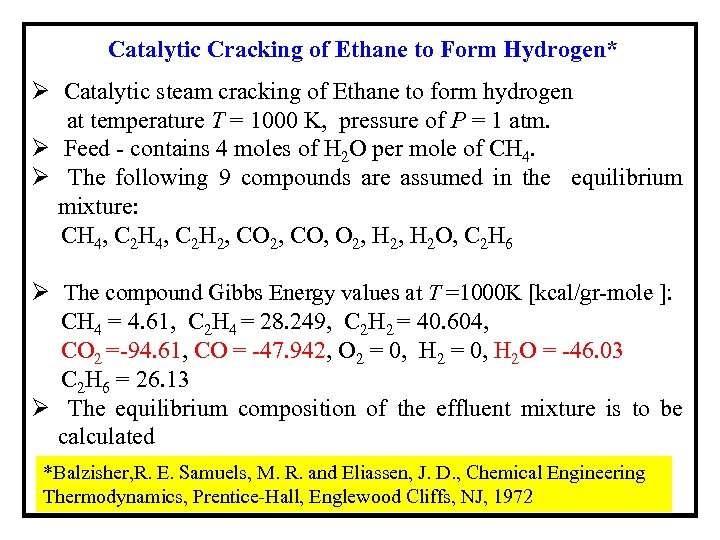Catalytic Cracking of Ethane to Form Hydrogen* Ø Catalytic steam cracking of Ethane to form hydrogen at temperature T = 1000 K, pressure of P = 1 atm. Ø Feed - contains 4 moles of H 2 O per mole of CH 4. Ø The following 9 compounds are assumed in the equilibrium mixture: CH 4, C 2 H 2, CO, O 2, H 2 O, C 2 H 6 Ø The compound Gibbs Energy values at T =1000 K [kcal/gr-mole ]: CH 4 = 4. 61, C 2 H 4 = 28. 249, C 2 H 2 = 40. 604, CO 2 =-94. 61, CO = -47. 942, O 2 = 0, H 2 O = -46. 03 C 2 H 6 = 26. 13 Ø The equilibrium composition of the effluent mixture is to be calculated *Balzisher, R. E. Samuels, M. R. and Eliassen, J. D. , Chemical Engineering Thermodynamics, Prentice-Hall, Englewood Cliffs, NJ, 1972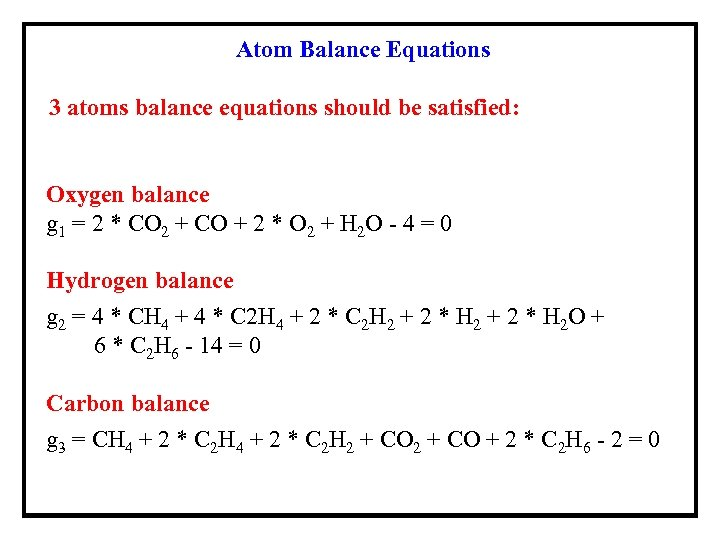Atom Balance Equations 3 atoms balance equations should be satisfied: Oxygen balance g 1 = 2 * CO 2 + CO + 2 * O 2 + H 2 O - 4 = 0 Hydrogen balance g 2 = 4 * CH 4 + 4 * C 2 H 4 + 2 * C 2 H 2 + 2 * H 2 O + 6 * C 2 H 6 - 14 = 0 Carbon balance g 3 = CH 4 + 2 * C 2 H 2 + CO + 2 * C 2 H 6 - 2 = 0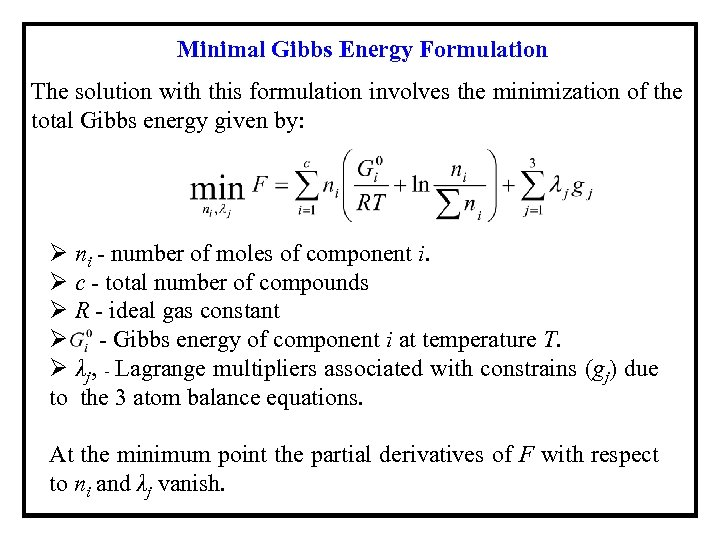Minimal Gibbs Energy Formulation The solution with this formulation involves the minimization of the total Gibbs energy given by: Ø ni - number of moles of component i. Ø c - total number of compounds Ø R - ideal gas constant Ø - Gibbs energy of component i at temperature T. Ø λj, - Lagrange multipliers associated with constrains (gj) due to the 3 atom balance equations. At the minimum point the partial derivatives of F with respect to ni and λj vanish.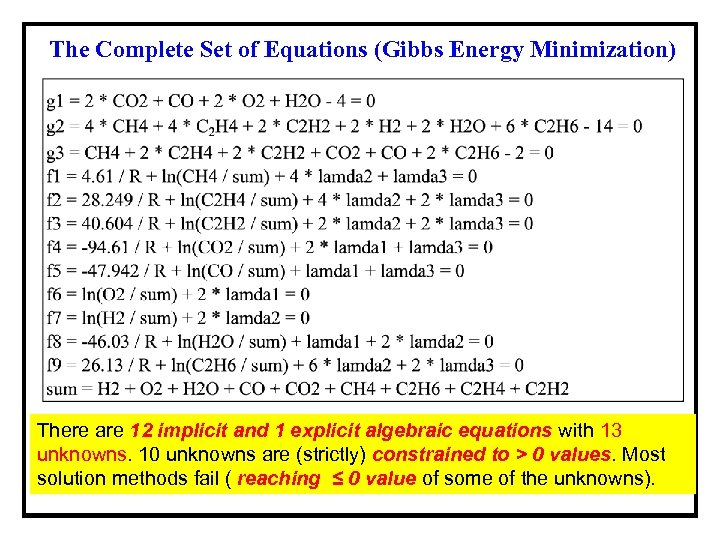The Complete Set of Equations (Gibbs Energy Minimization) There are 12 implicit and 1 explicit algebraic equations with 13 unknowns. 10 unknowns are (strictly) constrained to > 0 values. Most solution methods fail ( reaching ≤ 0 value of some of the unknowns).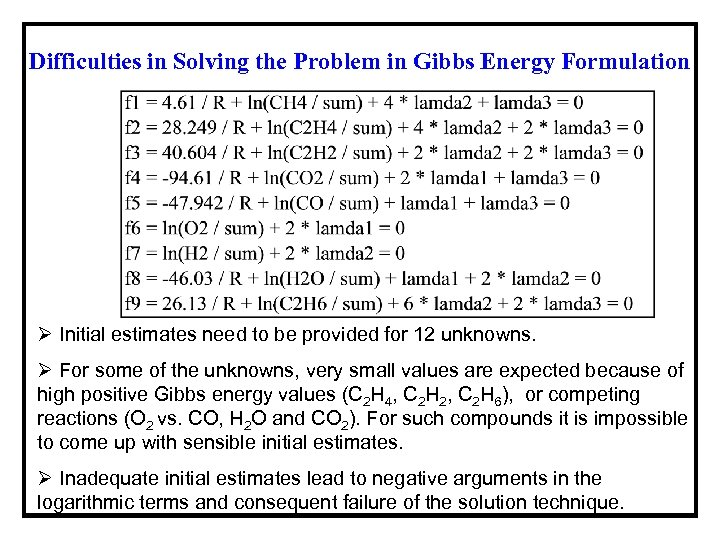Difficulties in Solving the Problem in Gibbs Energy Formulation Ø Initial estimates need to be provided for 12 unknowns. Ø For some of the unknowns, very small values are expected because of high positive Gibbs energy values (C 2 H 4, C 2 H 2, C 2 H 6), or competing reactions (O 2 vs. CO, H 2 O and CO 2). For such compounds it is impossible to come up with sensible initial estimates. Ø Inadequate initial estimates lead to negative arguments in the logarithmic terms and consequent failure of the solution technique.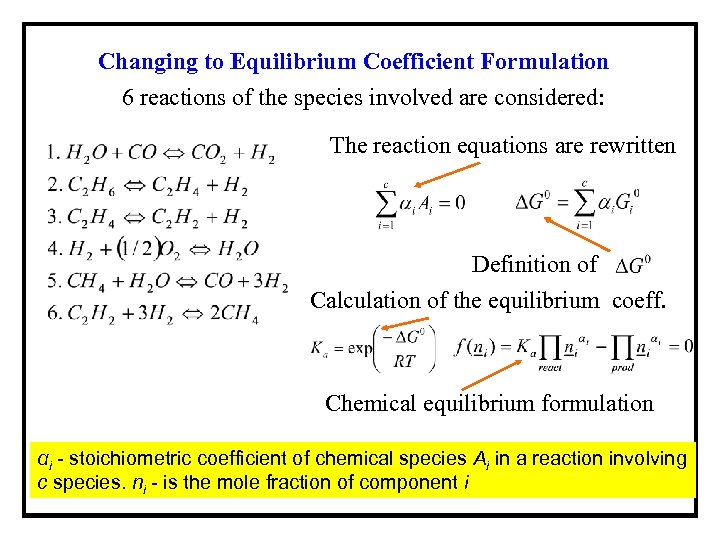Changing to Equilibrium Coefficient Formulation 6 reactions of the species involved are considered: The reaction equations are rewritten Definition of Calculation of the equilibrium coeff. Chemical equilibrium formulation αi - stoichiometric coefficient of chemical species Ai in a reaction involving c species. ni - is the mole fraction of component i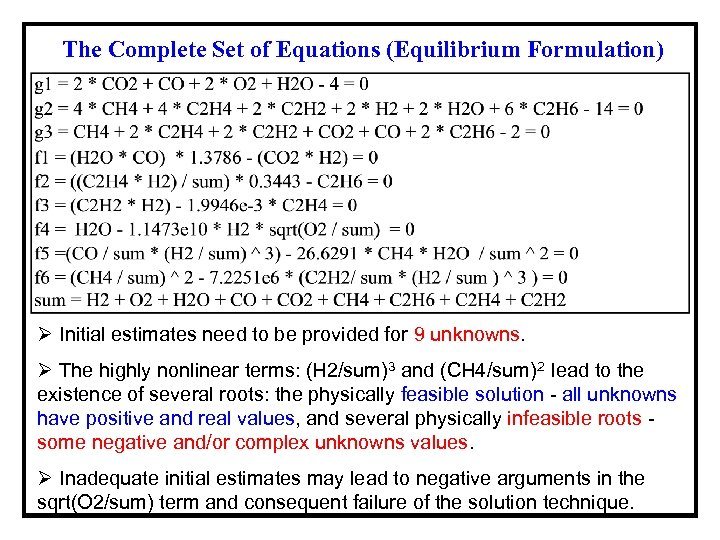The Complete Set of Equations (Equilibrium Formulation) Ø Initial estimates need to be provided for 9 unknowns. Ø The highly nonlinear terms: (H 2/sum)3 and (CH 4/sum)2 lead to the existence of several roots: the physically feasible solution - all unknowns have positive and real values, and several physically infeasible roots some negative and/or complex unknowns values. Ø Inadequate initial estimates may lead to negative arguments in the sqrt(O 2/sum) term and consequent failure of the solution technique.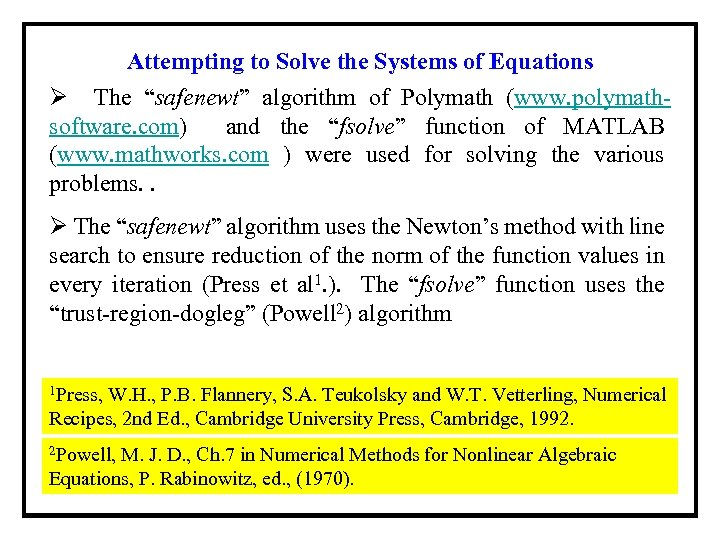Attempting to Solve the Systems of Equations Ø The “safenewt” algorithm of Polymath (www. polymathsoftware. com) and the “fsolve” function of MATLAB (www. mathworks. com ) were used for solving the various problems. . Ø The “safenewt” algorithm uses the Newton’s method with line search to ensure reduction of the norm of the function values in every iteration (Press et al 1. ). The “fsolve” function uses the “trust-region-dogleg” (Powell 2) algorithm 1 Press, W. H. , P. B. Flannery, S. A. Teukolsky and W. T. Vetterling, Numerical Recipes, 2 nd Ed. , Cambridge University Press, Cambridge, 1992. 2 Powell, M. J. D. , Ch. 7 in Numerical Methods for Nonlinear Algebraic Equations, P. Rabinowitz, ed. , (1970).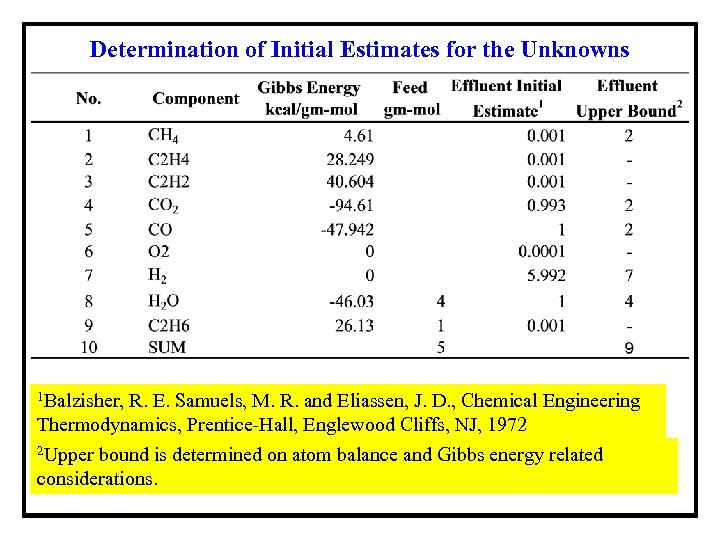Determination of Initial Estimates for the Unknowns 1 Balzisher, R. E. Samuels, M. R. and Eliassen, J. D. , Chemical Engineering Thermodynamics, Prentice-Hall, Englewood Cliffs, NJ, 1972 2 Upper bound is determined on atom balance and Gibbs energy related considerations.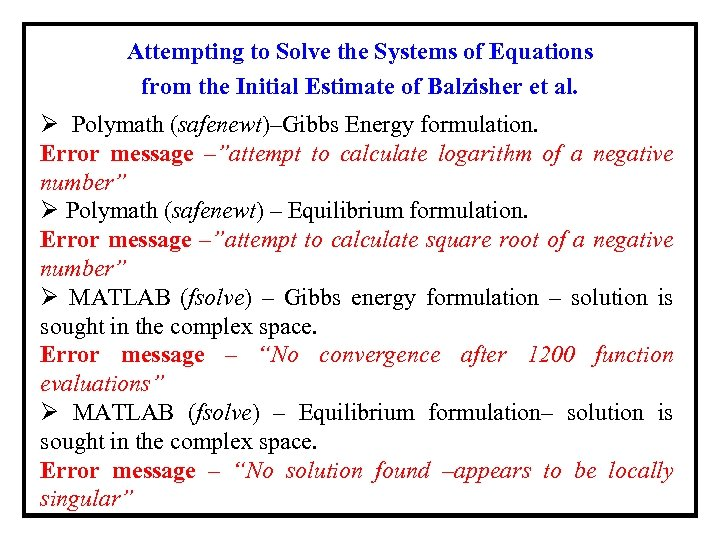Attempting to Solve the Systems of Equations from the Initial Estimate of Balzisher et al. Ø Polymath (safenewt)–Gibbs Energy formulation. Error message –”attempt to calculate logarithm of a negative number” Ø Polymath (safenewt) – Equilibrium formulation. Error message –”attempt to calculate square root of a negative number” Ø MATLAB (fsolve) – Gibbs energy formulation – solution is sought in the complex space. Error message – “No convergence after 1200 function evaluations” Ø MATLAB (fsolve) – Equilibrium formulation– solution is sought in the complex space. Error message – “No solution found –appears to be locally singular”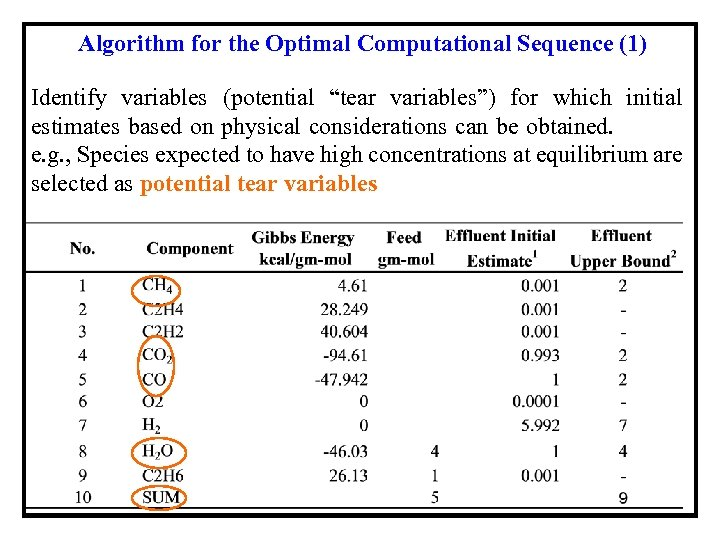Algorithm for the Optimal Computational Sequence (1) Identify variables (potential “tear variables”) for which initial estimates based on physical considerations can be obtained. e. g. , Species expected to have high concentrations at equilibrium are selected as potential tear variables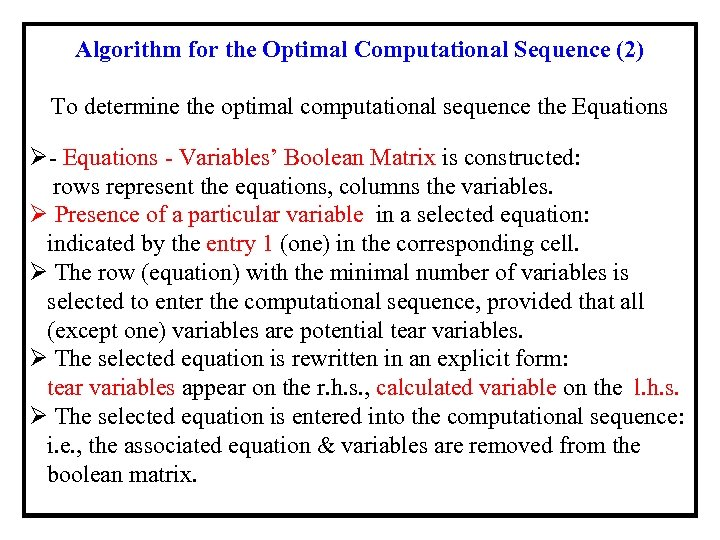Algorithm for the Optimal Computational Sequence (2) To determine the optimal computational sequence the Equations Ø- Equations - Variables’ Boolean Matrix is constructed: rows represent the equations, columns the variables. Ø Presence of a particular variable in a selected equation: indicated by the entry 1 (one) in the corresponding cell. Ø The row (equation) with the minimal number of variables is selected to enter the computational sequence, provided that all (except one) variables are potential tear variables. Ø The selected equation is rewritten in an explicit form: tear variables appear on the r. h. s. , calculated variable on the l. h. s. Ø The selected equation is entered into the computational sequence: i. e. , the associated equation & variables are removed from the boolean matrix.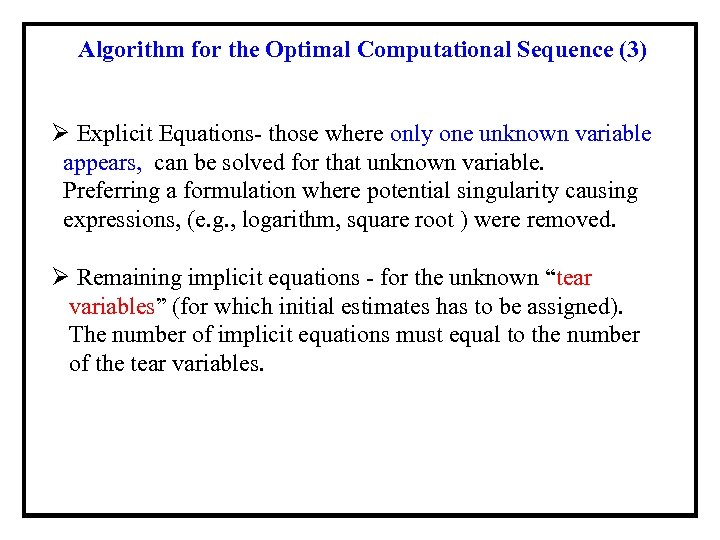Algorithm for the Optimal Computational Sequence (3) Ø Explicit Equations- those where only one unknown variable appears, can be solved for that unknown variable. Preferring a formulation where potential singularity causing expressions, (e. g. , logarithm, square root ) were removed. Ø Remaining implicit equations - for the unknown “tear variables” (for which initial estimates has to be assigned). The number of implicit equations must equal to the number of the tear variables.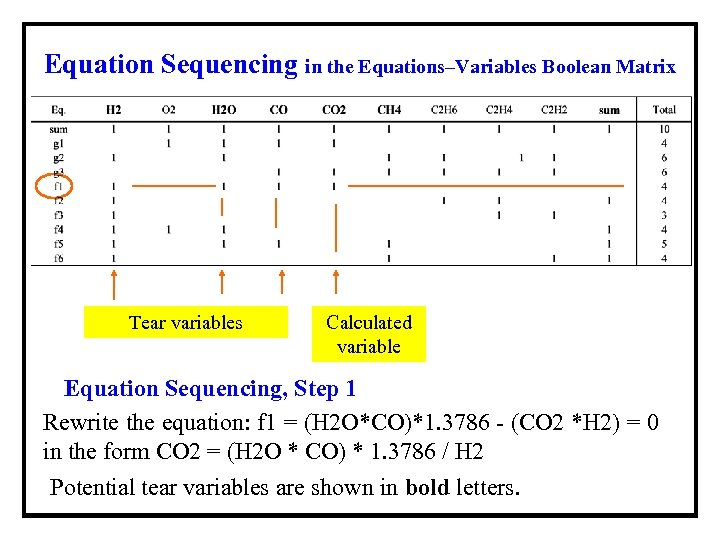Equation Sequencing in the Equations–Variables Boolean Matrix Tear variables Calculated variable Equation Sequencing, Step 1 Rewrite the equation: f 1 = (H 2 O*CO)*1. 3786 - (CO 2 *H 2) = 0 in the form CO 2 = (H 2 O * CO) * 1. 3786 / H 2 Potential tear variables are shown in bold letters.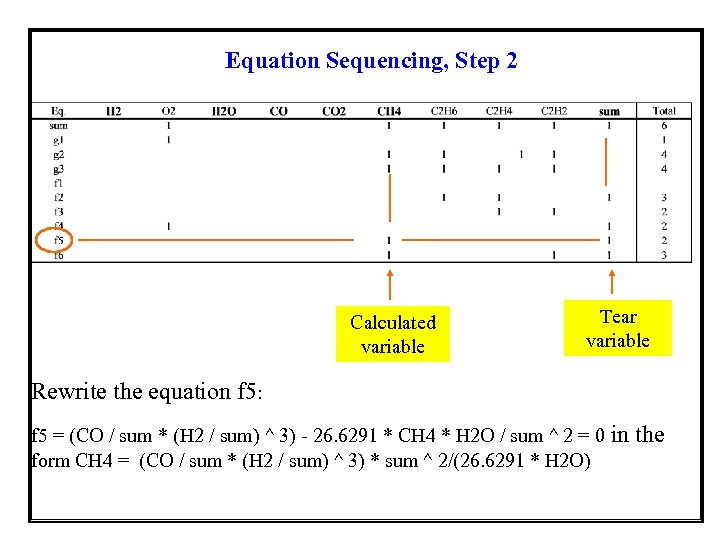Equation Sequencing, Step 2 Calculated variable Tear variable Rewrite the equation f 5: f 5 = (CO / sum * (H 2 / sum) ^ 3) - 26. 6291 * CH 4 * H 2 O / sum ^ 2 = 0 form CH 4 = (CO / sum * (H 2 / sum) ^ 3) * sum ^ 2/(26. 6291 * H 2 O) in the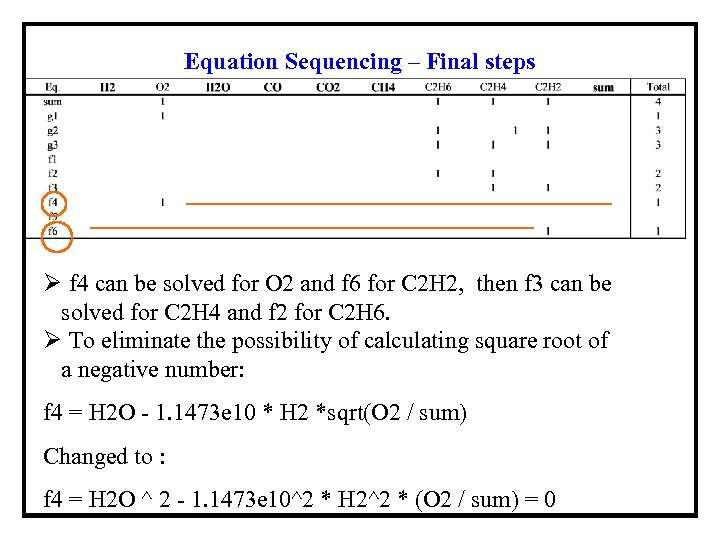Equation Sequencing – Final steps Ø f 4 can be solved for O 2 and f 6 for C 2 H 2, then f 3 can be solved for C 2 H 4 and f 2 for C 2 H 6. Ø To eliminate the possibility of calculating square root of a negative number: f 4 = H 2 O - 1. 1473 e 10 * H 2 *sqrt(O 2 / sum) Changed to : f 4 = H 2 O ^ 2 - 1. 1473 e 10^2 * H 2^2 * (O 2 / sum) = 0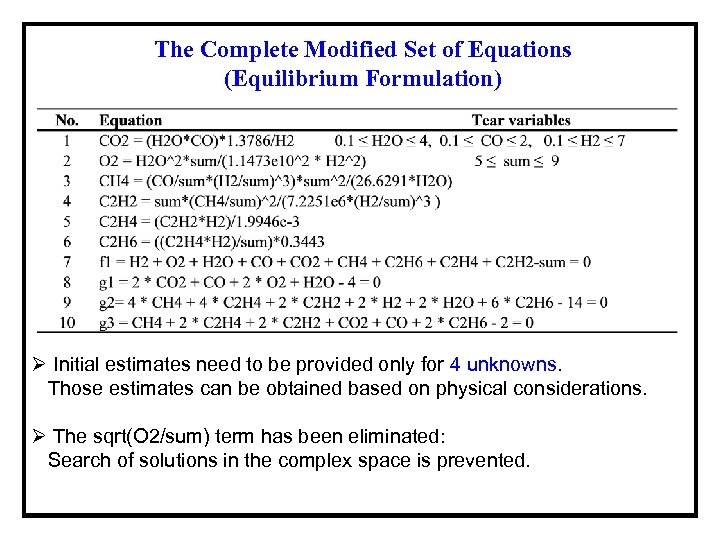The Complete Modified Set of Equations (Equilibrium Formulation) Ø Initial estimates need to be provided only for 4 unknowns. Those estimates can be obtained based on physical considerations. Ø The sqrt(O 2/sum) term has been eliminated: Search of solutions in the complex space is prevented.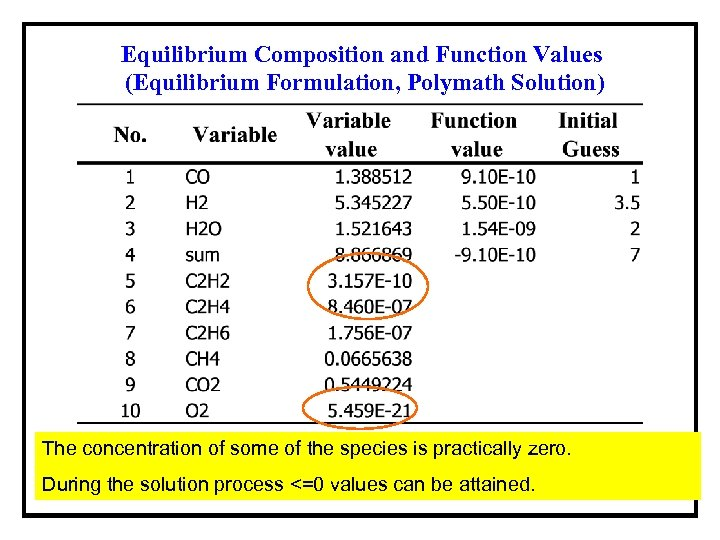Equilibrium Composition and Function Values (Equilibrium Formulation, Polymath Solution) The concentration of some of the species is practically zero. During the solution process <=0 values can be attained.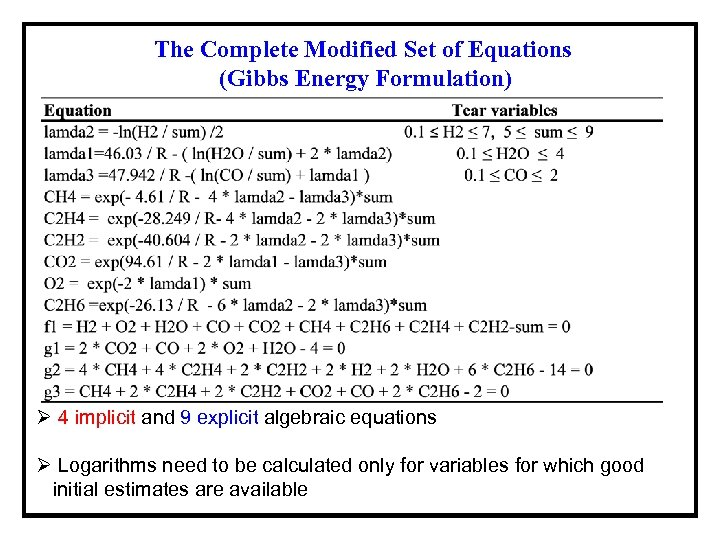The Complete Modified Set of Equations (Gibbs Energy Formulation) Ø 4 implicit and 9 explicit algebraic equations Ø Logarithms need to be calculated only for variables for which good initial estimates are available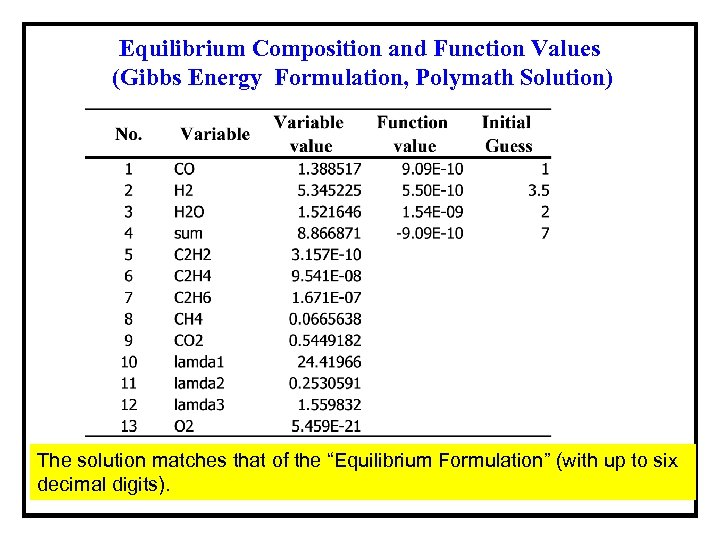Equilibrium Composition and Function Values (Gibbs Energy Formulation, Polymath Solution) The solution matches that of the “Equilibrium Formulation” (with up to six decimal digits).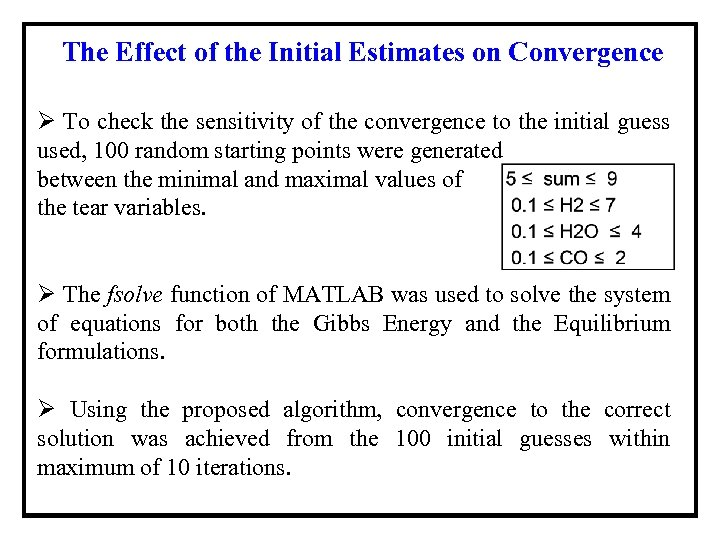The Effect of the Initial Estimates on Convergence Ø To check the sensitivity of the convergence to the initial guess used, 100 random starting points were generated between the minimal and maximal values of the tear variables. Ø The fsolve function of MATLAB was used to solve the system of equations for both the Gibbs Energy and the Equilibrium formulations. Ø Using the proposed algorithm, convergence to the correct solution was achieved from the 100 initial guesses within maximum of 10 iterations.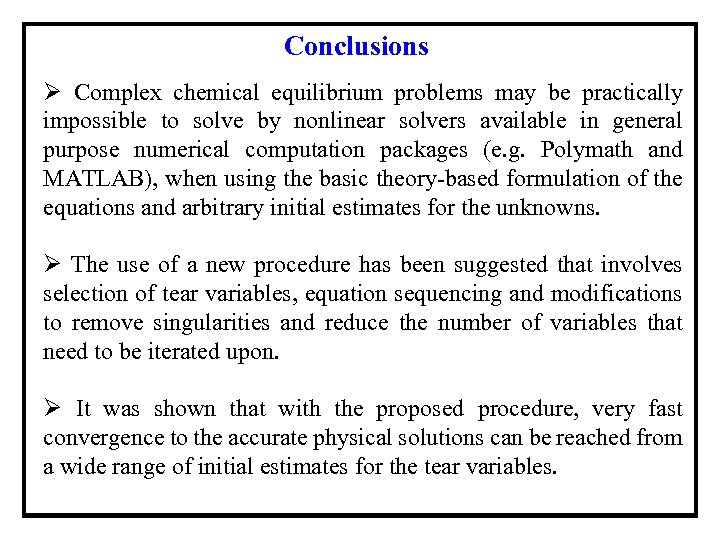Conclusions Ø Complex chemical equilibrium problems may be practically impossible to solve by nonlinear solvers available in general purpose numerical computation packages (e. g. Polymath and MATLAB), when using the basic theory-based formulation of the equations and arbitrary initial estimates for the unknowns. Ø The use of a new procedure has been suggested that involves selection of tear variables, equation sequencing and modifications to remove singularities and reduce the number of variables that need to be iterated upon. Ø It was shown that with the proposed procedure, very fast convergence to the accurate physical solutions can be reached from a wide range of initial estimates for the tear variables.